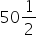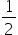Mathematics
Easy

Question

# Convertg into milligrams.

## The correct answer is: 50500

### We know, 1 g = 1000mgThen,g = 50 × 1000 +× 1000= 50000 + 500= 50500 mg

One g is equal to 1000 mg. therefore, (2 x 50 +1)/2 g = 101/2 g = 101/2  x 1000 mg = 50,500 mg

### Related Questions to study#### With Turito Foundation.#### Get an Expert Advice From Turito.